# High Frequency Engineering | Transit Time & Skin Effect Basics

By Aman Deep, from Shri Govindram Seksaria Institute of Technology and Science

6956

Consider a simple circuit connected to AC source, transmission line and a load. The load may contain the passive components like capacitor, resistor or inductor. Given below is a simple setup that we shall be using for our analysis.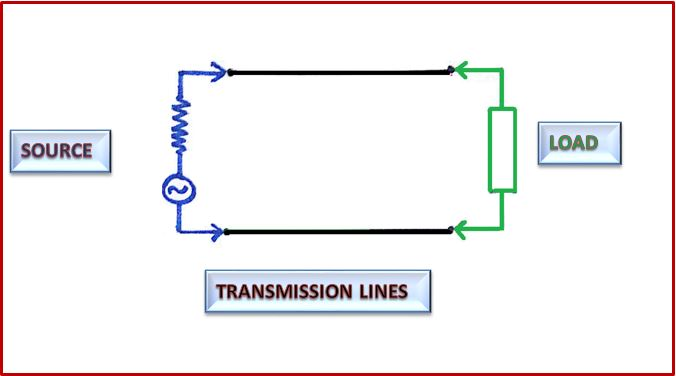It contains 3 components,

1. Source
The source is shown in blue in the figure.  It gives sinusoidal input voltage to the circuit of a particular frequency. It has an internal resistance associated with it called source resistance.
2. Transmission Lines
These are the connecting wires for the current to flow in them. They are shown in black in the figure.
Load is shown in green in the fig. It may contain combination of passive elements like resistor, capacitor or inductor. It’s effective resistance when connected to an AC circuit is called impedance. Load in our daily life are the various appliances running around us like fans, bulbs, refrigerator etc.

The source gives voltage in the sinusoidal form of a particular amplitude and frequency. Keep in mind that sine and cos, both are sinusoidal waves.

## Wavelength

Wavelength of the sinusoidal wave is defined as the distance between two successive crest of a wave. It is denoted by ‘Λ’.

## Frequency

Frequency is defined as the no of cycles a wave can make in 1 sec. It is measured in Hertz. Following figure shall make our idea more clear about the frequency. It is denoted by ‘f’.

# Relation between wavelength and frequency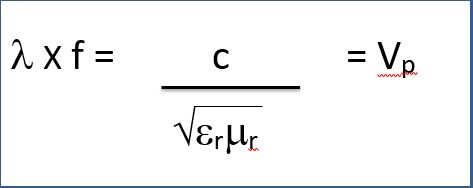Where;

• Λ    =  Wavelength
• f     =  frequency
• c     =  Speed of light (3 X 108)
• Vp    =  Phase velocity
• er     =  Relative permittivity
• ur   =  Relative permiability

The above relation gives us the relation between the frequency and the wavelength of the input sinusoidal signal. We observe that frequency and wavelength are inversely proportional to each other.

## So we define high frequency as follows

When the length of the circuit is comparable to the wavelength of the input sinusoidal wave, then frequency corresponding to that wavelength is considered as high frequency.
We shall now look into the concept of transit time effect.

# Transit Time Effect

Consider a simple circuit connected to AC source, transmission line and a load.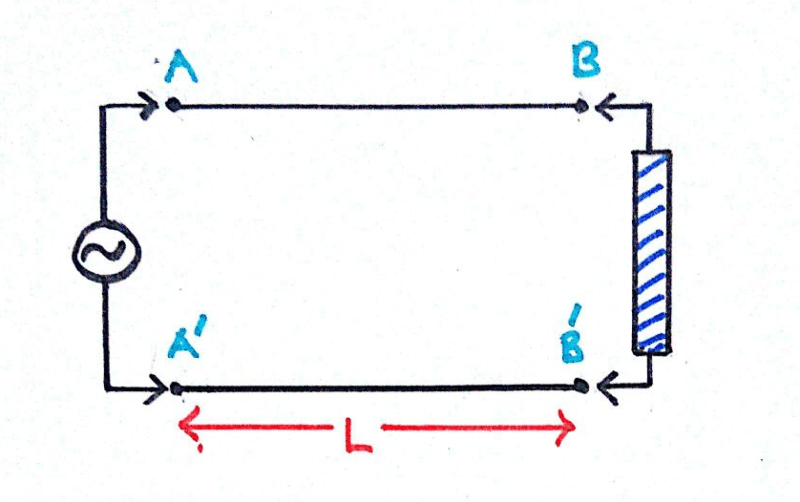We apply a sinusoidal voltage of frequency ‘f’ and hence time period 1/f.

Let at some instant of time the voltage across AA’ is Vx. It is a fact that no signal can travel with infinite speed in a physical system. Hence this voltage Vx does not occur at BB’ at the same instant. It will appear at BB’ after some finite delay of time. This finite delay is called transit time of the signal for the length of the line i.e. time taken for the voltage applied at one end to appear at the other end.

Transit time is given by;

#### tr = L/v

Where :

• tr = Transit Time
• L= Length of the conductor (Transmission line)
• V= Velocity

Thus the transit time is the time after which the voltage at A appears at B.

At t=0;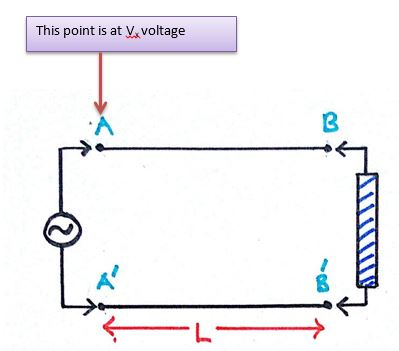When this voltage appears at BB’, the voltage at AA’ does does not remain Vx as it was varying with time. Let us assume it becomes Vy.

At t= tr (Transit time)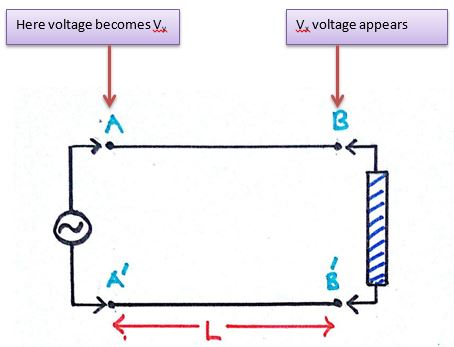Thus a potential difference is developed between the points A and B. No matter how small the length of the conducting wire, some amount of potential drop will always be there. This is called the transit time effect.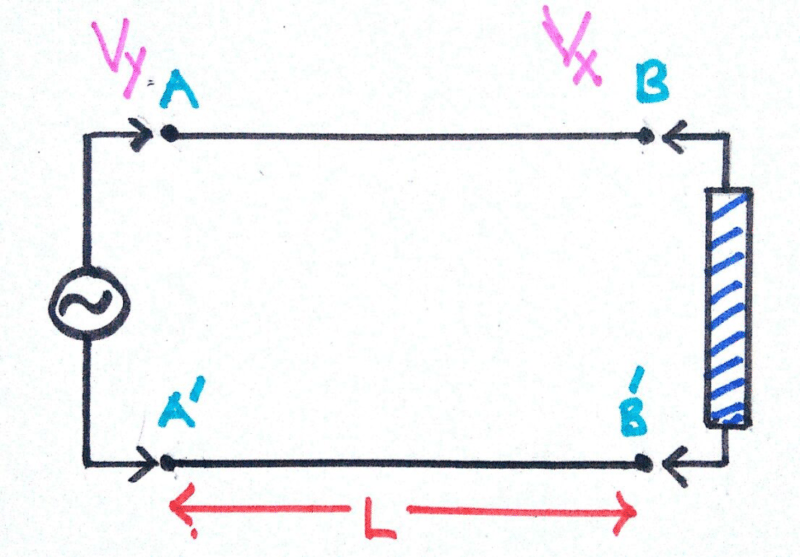The transit time effect can be neglected if the potential drop between A and B is very small. The transit time effect can be neglected if the potential drop between A and B is very small. So essentially what we say is that if the Transit time is much smaller compared to the time period of the signal then the transit time effects can be neglected.